# 大神是怎样用函数式JavaScript计算数组平均值的

+关注继续查看

reduce()来计算数组的平均值是一个常用的模式。代码看起来非常简单，不过在计算最终结果之前你需要做两个准备工作：

• 数组的长度
• 数组所有元素之和

function average(nums) {
return nums.reduce((a, b) => a + b) / nums.length;
}

## 问题提出

const victorianSlang = [
{
term: "doing the bear",
found: true,
popularity: 108
},
{
term: "katterzem",
found: false,
popularity: null
},
{
term: "bone shaker",
found: true,
popularity: 609
},
{
term: "smothering a parrot",
found: false,
popularity: null
},
{
term: "damfino",
found: true,
popularity: 232
},
{
term: "rain napper",
found: false,
popularity: null
},
{
term: "donkey’s breakfast",
found: true,
popularity: 787
},
{
term: "rational costume",
found: true,
popularity: 513
},
{
term: "mind the grease",
found: true,
popularity: 154
}
];

## 1. for 循环

let popularitySum = 0;
let itemsFound = 0;
const len = victorianSlang.length;
let item = null;
for (let i = 0; i < len; i++) {
item = victorianSlang[i];
if (item.found) {
popularitySum = item.popularity + popularitySum;
itemsFound = itemsFound + 1;
}
}
const averagePopularity = popularitySum / itemsFound;
console.log("Average popularity:", averagePopularity);

1. 初始化polularitySumitemsFound变量。popularitySum记录总的流行度值，itemsFound记录我们已经找到的所有的条目；
2. 初始化lenitem来帮助我们遍历数组；
3. for 循环每一次增加i的值，直到循环n次；
4. 在循环中，我们每次取出当前索引位置的条目vitorianSlang[i]
6. 如果在，获取popularity并累加到popularitySum
7. 并递增itemsFound
8. 最后，用popularitySum除以itemsFound来计算平均值。

## 2. 简单模式: filter, map 和 sum

1. 使用fitler()找到那些在 Google Books 中的条目;
2. 使用map()获取流行度；
3. 使用reuduce()来计算总的流行度；
4. 计算平均值。

// 辅助函数
// ----------------------------------------------------------------------------
function isFound(item) {
return item.found;
}

function getPopularity(item) {
return item.popularity;
}

return runningTotal + popularity;
}

// 计算
// ----------------------------------------------------------------------------

// 找出所有isFound为true的条目
const foundSlangTerms = victorianSlang.filter(isFound);

// 从条目中获取流行度值，返回为数组
const popularityScores = foundSlangTerms.map(getPopularity);

// 求和

// 计算平均值
const averagePopularity = scoresTotal / popularityScores.length;
console.log("Average popularity:", averagePopularity);

## 3. 简单模式 II: 记录多个累加值

// 辅助函数
// ---------------------------------------------------------------------------------
function isFound(item) {
return item.found;
}

function getPopularity(item) {
return item.popularity;
}

// 我们使用一个对象来记录总的流行度和条目的总数
function addScores({ totalPopularity, itemCount }, popularity) {
return {
totalPopularity: totalPopularity + popularity,
itemCount: itemCount + 1
};
}

// 计算
// ---------------------------------------------------------------------------------

const initialInfo = { totalPopularity: 0, itemCount: 0 };
const popularityInfo = victorianSlang
.filter(isFound)
.map(getPopularity)

const { totalPopularity, itemCount } = popularityInfo;
const averagePopularity = totalPopularity / itemCount;
console.log("Average popularity:", averagePopularity);

## 4. point-free 式函数组合

// 辅助函数
// ----------------------------------------------------------------------------
const filter = p => a => a.filter(p);
const map = f => a => a.map(f);
const prop = k => x => x[k];
const reduce = r => i => a => a.reduce(r, i);
const compose = (...fns) => arg => fns.reduceRight((arg, fn) => fn(arg), arg);

// The blackbird combinator.
// See: https://jrsinclair.com/articles/2019/compose-js-functions-multiple-parameters/
const B1 = f => g => h => x => f(g(x))(h(x));

// 计算
// ----------------------------------------------------------------------------

// 求和函数
const sum = reduce((a, i) => a + i)(0);

// 计算数组长度的函数
const length = a => a.length;

// 除法函数
const div = a => b => a / b;

// 我们使用compose()来将函数组合起来
// compose()的参数你可以倒着读，来理解程序的含义
const calcPopularity = compose(
B1(div)(sum)(length),
map(prop("popularity")),
filter(prop("found"))
);

const averagePopularity = calcPopularity(victorianSlang);
console.log("Average popularity:", averagePopularity);

// All the lines below are equivalent:
const avg1 = B1(div)(sum)(length);
const avg2 = arr => div(sum(arr))(length(arr));
const avg3 = arr => sum(arr) / length(arr);
const avg4 = arr => arr.reduce((a, x) => a + x, 0) / arr.length;

## 5. 终极优化: 一次计算出结果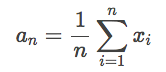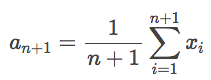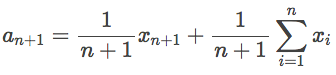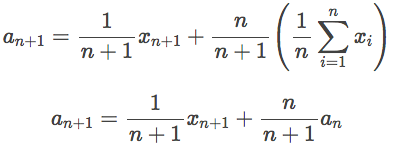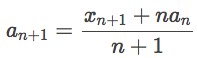// 求平均值
function averageScores({ avg, n }, slangTermInfo) {
if (!slangTermInfo.found) {
return { avg, n };
}
return {
avg: (slangTermInfo.popularity + n * avg) / (n + 1),
n: n + 1
};
}

const initialVals = { avg: 0, n: 0 };
const averagePopularity = victorianSlang.reduce(averageScores, initialVals).avg;
console.log("Average popularity:", averagePopularity);

### 版权声明javascript使用栈结构将中缀表达式转换为后缀表达式并计算值
1.概念 　　你可能听说过表达式，a+b，a+b*c这些，但是前缀表达式，前缀记法，中缀表达式，波兰式，后缀表达式，后缀记法，逆波兰式这些都是也是表达式。 　　a+b，a+b*c这些看上去比较正常的是中缀表达式，就是运算符放在两个操作数之间。
1158 01150 0javascript 表达式、括号、常用函数和jquery库怎么样实现的分析
（一）javascript表达式 表达式是什么？表达式是对变更进行赋值、更改或计算等操作的语句。它是变量、常量、操作符的综合。根据操作符的不类型，可以分为字符操作表达式、赋值表达式、逻辑表达式、关系表达式、自增自减表达式、位表达式等。
740 0【Java8新特性】还没搞懂函数式接口？赶快过来看看吧！
Java8中内置了一些在开发中常用的函数式接口，极大的提高了我们的开发效率。那么，问题来了，你知道都有哪些函数式接口吗？
61 0JavaScript创建对象（四）——组合使用构造函数和原型模式

873 0javascript 一个关于时间排序的算法（一个页面多个倒计时排序）

882 0969 0JavaScript计算字符串中每个字符出现的次数
955 02018年杭州云栖大会-企业办公数据处理和分发（函数计算篇）

1414 08 0+关注
fundebug

228

1

OceanBase 入门到实战教程